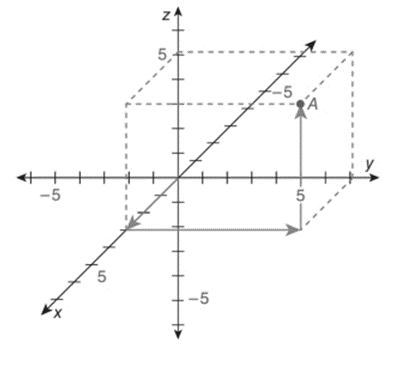Chapter 10.6, Problem 1EElementary Geometry For College St...

7th Edition
Alexander + 2 others
ISBN: 9781337614085

Solutions

Chapter
SectionElementary Geometry For College St...

7th Edition
Alexander + 2 others
ISBN: 9781337614085
Textbook Problem

In the Cartesian coordinate system below, name the ordered triple x ,   y ,   z represented by point A. Also, plot the point B 5 ,   6 ,   4 .To determine

To name:

The ordered triple found in the given figure.

Explanation

The given figure is shown below.

In a three dimensional Cartesian coordinate system, x-axis moves forward and backward.

Forward means positive coordinate.

Backward means negative coordinate.

y-axis moves right and left.

Right means positive coordinate.

Left means negative coordinate.

z-axis moves upward and downward.

Upward means positive coordinate.

Downward means negative coordinate.

In the given figure,

Beginning from the origin, x-axis moves 3 units forward, y-axis moves 7 units to theright and z-axis moves 5 units upwards to arrive at the given point A.

Hence, the ordered triple is A3, 7, 5

Still sussing out bartleby?

Check out a sample textbook solution.

See a sample solution

The Solution to Your Study Problems

Bartleby provides explanations to thousands of textbook problems written by our experts, many with advanced degrees!

Get Started

Evaluate the integral. xsin3xdx

Calculus (MindTap Course List)

Which of the following three graphs is the graph of ? Explain your choice.

Mathematical Applications for the Management, Life, and Social Sciences

Convert the expressions in Exercises 6584 to power form. x3

Finite Mathematics and Applied Calculus (MindTap Course List)

2,339+118+3,650+8,770+81+6=

Contemporary Mathematics for Business & Consumers